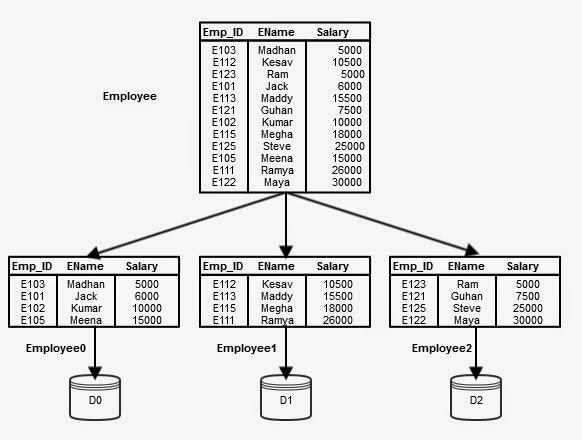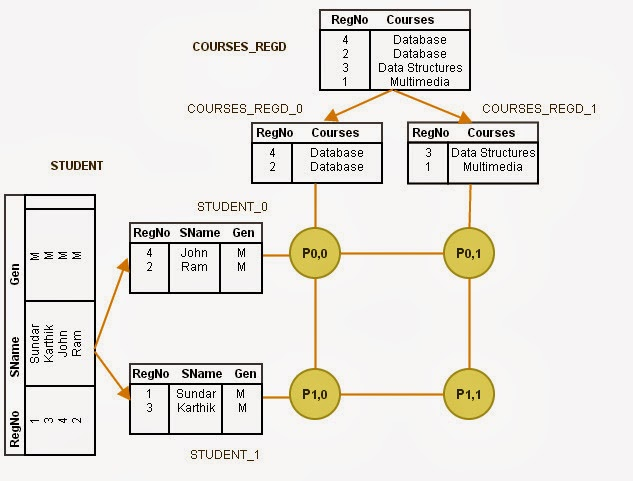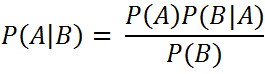`  I request GUEST WRITERS for this web site. Anyone interested please contact me at saravu2k2013@gmail.com Thanks  `
Showing posts with label Intraoperation Parallelism. Show all posts
Showing posts with label Intraoperation Parallelism. Show all posts

## How do we perform Duplicate data elimination, Projection, and Aggregation in parallel in parallel database system?

Assume that the table is partitioned and stored in disks D0, D1, …, Dn-1 with processors P0, P1, …, Pn-1. For example, consider the following figure where the table Employee is partitioned used Round-robin partitioning technique.Figure 1 - Partitioning Employee table

### Duplication elimination:

Duplicate elimination is about removing duplicate values or neglecting repeated values that are stored in an attribute for various reasons; For example, in one or all of the following situations we need duplicate elimination;

• When we would like to count the number of unique values present in a table under a particular attribute.
• When we need to retrieve the values and present only the unique values of an attribute.
• When we need to join two tables, etc.
The main approach used for duplicate elimination is by sorting the data on the attribute where in the duplicate values to be removed.
Duplicate elimination can be achieved in the following two ways in parallel database;
1. During parallel sort, if we find any repeated values while partitioning, those can be discarded immediately. (This method is for tables that are not partitioned). For example, in Figure 1, while you partition the data, you send tuples to different partitions based on the partition attribute and conditions. Suppose that we are partitioning on Salary attribute. During the process, if you find any of the repeated values which are already send into a partition, you can discard those values if they repeat. In our example, salary value 5000 occurs in two records. Hence, one can be accepted and the other can be discarded.
2. We can partition the table into many partitions (using range or hash partitioning), and instruct all the processors to sort the data locally and remove the duplicates. (This works only for the data that are hash or range partitioned on the duplicate elimination attribute)

### Projection:

Projection means selection of one or more attributes from a table with the records stored in them. This operation can be parallelized as follows;
1. Projection without duplicate elimination: while you read data into various disks during partitioning, you can project the required columns.
2. Projection with duplicate elimination: any of the techniques suggested in Duplicate elimination section above can be used.

### Aggregation:

Aggregation operation involves finding the count of records, sum of values stored in an attribute, minimum or maximum value of all the values stored in an attribute, and average value of all the attribute values. This operation basically needs grouping. That is, for example, we can find the sum of salary for all the records of Employee table, or we can find sum of salary with some filter conditions. In the first case, all the records of Employee table come under one group. In the later case, we choose the group based on the conditions included.

## How would we parallelize the SELECTION operation in an SQL query?

• Assume that the table is partitioned and stored in disks D0, D1, …, Dn-1 with processors P0, P1, …, Pn-1. For example, consider the following figure where the table Employee is partitioned using Round-robin partitioning technique.Figure 1 - Partitioned table Employee
• Recall the types of queries based on data access. Basically we have three types;
• Point queries – In point queries, the WHERE conditions are specified like “attribute_name = value” format.
• Range queries – In range queries, the WHERE conditions are specified like “attribute_name >= value AND attribute_name <= value”, or “attribute_name BETWEEN value1 and value 2”.
• Scanning the entire relation – In queries where the WHERE condition involves non-key attributes, then the system has to look for all the data in the specified table. This is called the relation scan.
With this information let us consider the following general syntax of any SQL select query;

SELECT list_of_attributes FROM table_name WHERE condition;

This query can be parallelized based on the condition given in the WHERE clause.

CASE 1: If the condition is of the form “a = value”, then;
If the given table is partitioned on a, then we need to execute the selection operation in only single processor where in the given attribute is partitioned.
CASE 2: If the condition is of the form “value1 <= a <= value2” (i.e, a falls in a range), then;
If the given relation is range partitioned on a, then we need to perform selection at all the processors wherever the range overlaps with the given range value1 to value2.
CASE 3: In all the other cases, the selection performed in parallel in all the processors. That is, for all the other type of queries, the selection can be done in parallel in all the processors, because, the data are partitioned over several processors.

As an example for CASE 3, let us assume a query which requests information from a table with a non-key attribute in the WHERE clause condition. Refer the Employee table in Figure 1.
SELECT * FROM emp WHERE ename = ‘Kumar’;
The query involves a condition on name attribute and we don’t create an index or key on name attribute as there are more such names. Hence, this query needs to examine all records of Employee table to give the final result. So, we can distribute the query to all the processors wherever Employee table's partitions are stored, and execute the query in parallel on all the processors. According to the example in Figure 1, Processors P0, P1, and P2 have to execute the query locally, even though the data available at D0 alone. The final result is generated from the local results of these processors. This way the parallelism for SELECTION operation can be achieved.
The same (CASE 3) is applicable for other type of actions like,

• If the table is partitioned based on an attribute other than the WHERE condition attribute,
• If the table is range partitioned on an attribute other than the range attribute specified in the query,
• If the table is partitioned on one attribute and the query involves one more attribute with and condition, etc.

#### Point to note:

• According to CASE 3, it means, we can achieve parallelism by exploiting all the processors.
• According to CASE 1, only one processor has to execute and it is known initially. That means, the other processors can effectively be used for executing other queries at that time.
• According to CASE 2, only few processors where the range of values stored need to execute. The other processors can be utilized for executing other queries.

## Fragment and Replicate Join

#### Introduction

All of us know about different types of joins in terms of the nature of the join conditions and the operators used for join. They are Equi-join and Non-Equi Join. Equi-join is performed through checking an equality condition between different joining attributes of different tables. Non-equi join is performed through checking an inequality condition between joining attributes.
Equi-join is of the form,
SELECT columns FROM list_of_tables WHERE table1.column = table2.column;
whereas, Non-equi join is of the form,
SELECT columns FROM list_of_tables WHERE table1.column < table2.column;
(Or, any other operators >, <>, <=, >= etc. in the place of < in the above example)

We have discussed Partitioned Join in the previous post, where we partitioned the relational tables that are to be joined, into equal partitions and we performed join on individual partitions locally at every processor. Partitioning the relations on the joining attribute and join them will work only for joins that involve equality conditions.
Clearly, joining the tables by partitioning will work only for Equi-joins or natural joins. For inequality joins, partitioning will not work. Consider a join condition as given below;
r$\bowtie$r.a>s.b s
In this non-equal join condition, the tuples (records) of r must be joined with records of s for all the records where the value of attribute r.a is greater than s.b. In other words, all records of r join with some records of s and vice versa. That is, one of the relations’ all records must be joined with some of the records of other relation. For clear example, see Non-equi join post.

#### What does fragment and replicate mean?

Fragment means partitioning a table either horizontally or vertically (Horizontal and Vertical fragmentation). Replicate means duplicating the relation, i.e, generating similar copies of a table. This join is performed by fragmenting and replicating the tables to be joined.

### (How does Asymmetric Fragment and Replicate Join work?)

It is a variant of Fragment and Replicate join. It works as follows;
1. The system fragments table r into n fragments such that r0, r1, r2, .., rn-1, where r is one of the tables that is to be joined and n represents the number of processors. Any partitioning technique, round-robin, hash or range partitioning could be used to partition the relation.
2. The system replicates the other table, say s into n processors. That is, the system generates n copies of s and sends to n processors.
3. Now we have, r0 and s in processor P0,  r1 and s in processor P1, r2 and s in processor P2, .., rn-1 and s in processor Pn-1. The processor Pi is performing the join locally on ri and s.
Figure 1 given below shows the process of Asymmetric Fragment-and-Replicate join (it may not be the appropriate example, but it clearly shows the process);Figure 1 - process of Asymetric Fragment and Replicate Parallel Join

#### Points to Note:

1. Non-equal join can be performed in parallel.
2. If one of the relations to be joined is already partitioned into n processors, this technique is best suited, because we need to replicate the other relation.
3. Unlike in Partitioned Join, any partitioning techniques can be used.
4. If one of the relations to be joined is very small, the technique performs better.

### (How does Fragment and Replicate Join work?)

It is the general case of Asymmetric Fragment-and-Replicate join technique. Asymmetric technique is best suited if one of the relations to be joined is small, and if it can fit into memory. If the relations that are to be joined are large, and the joins is non-equal then we need to use Fragment-and-Replicate Join. It works as follows;
1. The system fragments table r into m fragments such that r0, r1, r2, .., rm-1, and s into n fragments such that s0, s1, s2, .., sn-1 . Any partitioning technique, round-robin, hash or range partitioning could be used to partition the relations.
2. The values for m and n are chosen based on the availability of processor. That is, we need at least m*n processors to perform join.
3. Now we have to distribute all the partitions of r and s into available processors. And, remember that we need to compare every tuple of one relation with every tuple of other relation. That is the records of r0 partition should be compared with all partitions of s, and the records of partition s0 should be compared with all partitions of r. This must be done with all the partitions of r and s as mentioned above. Hence, the data distribution is done as follows;
a. As we need m*n processors, let us assume that we have processors P0,0, P0,1, …, P0,n-1, P1,0, P1,1, …, Pm-1,n-1. Thus, processor Pi,j performs the join of ri with sj.
b. To ensure the comparison of every partition of r with every other partition of s, we replicate ri with the processors, Pi,0, Pi,1, Pi,2, …, Pi,n-1, where 0, 1, 2, …, n-1 are partitions of s. This replication ensures the comparison of every ri with complete s.
c. To ensure the comparison of every partition of s with every other partition of r, we replicate si with the processors, P0,i, P1,i, P2,i, …, Pm-1,i, where 0, 1, 2, …, m-1 are partitions of r. This replication ensures the comparison of every si with complete r.
4. Pi,j computes the join locally to produce the join result.
Figure 2 given below shows the process of general case Fragment-and-Replicate join (it may not be the appropriate example, but it clearly shows the process);Figure 2 - process of general case Fragment-and-Replicate Join

#### Points to Note:

1. Asymmetric Fragment-and-replicate join is the special case of general case Fragment-and-replicate join, where n or m is 1, i.e, if one of the relation does not have partitions.

2. When compared to asymmetric technique, Fragment-and-replicate join reduces the size of the tables at every processor.

3. Any partitioning techniques can be used and any joining technique can be used as well.

4. Fragment-and-replicate technique suits both Equi-join and Non-equi join.

5. It involves higher cost in partitioning.

An appropriate example will be discussed later.

### Related Posts:

Intraoperation Parallelism

Partitioned Parallel Join
Partitioned Parallel Hash-Join
Parallel Nested Loop Join

 Try to learn something about everything and everything about something. Thomas Huxley

### Simple introduction to Naive Bayes classifier

Simple introduction to Naive Bayes classifier What is Naive Bayes Classifier? A Naive Bayes classifier is a probabilistic classifier ...